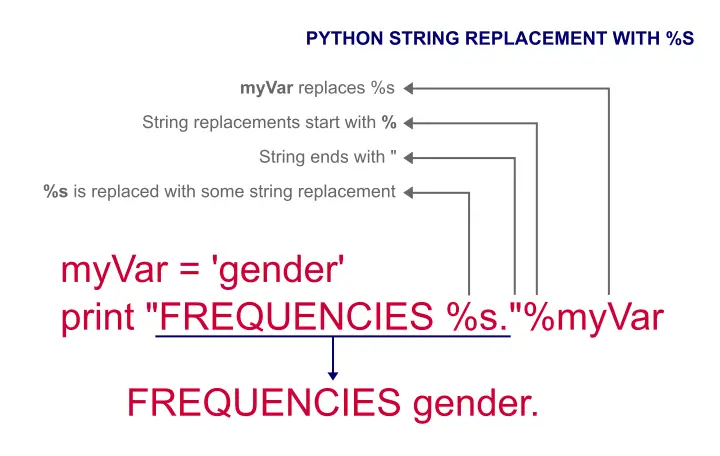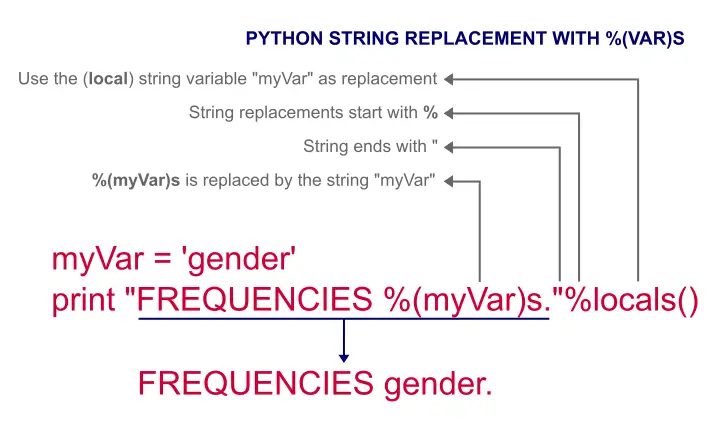SPSS TUTORIALS BASICS ANOVA REGRESSION FACTOR CORRELATION

# SPSS Python Text Replacement Tutorial

A common task in Python is building strings from other strings, especially if we're creating SPSS syntax. We could simply concatenate strings into larger strings but there's a nicer way: string replacements with the % sign.

Please note that the examples in this tutorial assume Python2.xPython2.x means any Python 2 version. Like so, Python2.x is short for Python 2.1 through Python2.7. and may not run in Python3.x.

## Python String Replacement with %s

 ```1 2 3 4 5 6 7 8 9 10 11 12 13``` *Replace text with concatenation. begin program. myVar = 'gender' print "FREQUENCIES " + myVar + "." end program. *Simple text replacement with %s. begin program. myVar = 'gender' print "FREQUENCIES %s."%myVar end program.

As we see, we just use `%s` in some string as a placeholder for some other string. The replacement for it is specified right after the ending quote, preceded by another % sign. The figure below zooms in on the code.## Python String Replacement with Locals()

Instead of using `%s` in some string, we may use `%(var)s`. Now, `var` is the name of some predefined Python variable known as a local variable. Using `%locals()` right after the ending quote tells Python to replace our placeholders with such local variables.

 ```1 2 3 4 5 6``` *Simple text replacement with locals(). begin program. myVar = 'gender' print "FREQUENCIES %(myVar)s."%locals() end program.## Multiple String Replacements in Python

We can use either `%s` or `%(var)s` more than once in one string but we can't mix them. The examples below -yielding identical results- use two replacements in one string with either method.

 ```1 2 3 4 5 6 7 8 9 10 11 12 13 14 15``` *Two text replacements with %s. begin program. oldVar = 'var1' newVar = 'top2_' + oldVar print "RECODE %s (1,2,3 = 0)(4,5 = 1)(ELSE = COPY) INTO %s."%(oldVar,newVar) end program. *Two text replacements with locals(). begin program. oldVar = 'var1' newVar = 'top2_' + oldVar print "RECODE %(oldVar)s (1,2,3 = 0)(4,5 = 1)(ELSE = COPY) INTO %(newVar)s."%locals() end program.

Note: if `%s` occurs twice, we must specify a tuple like `%(var1,var2)` right after our string. The first occurrence of `%s` is replaced by `var1` and the second by `var2`.

## Replace Text with String Method

So far, our examples replaced parts of strings with fixed, predefined other strings. But what if we need a Python string method instead? Example 1 (below) first applies `replace` to a string and then inserts it as usual.
An alternative is to replace `%s` directly with a string method as shown in example 2. Both examples yield identical results but use different approaches.

 ```1 2 3 4 5 6 7 8 9 10 11 12 13 14 15 16 17``` *Apply string function to local variable, then replace with locals(). begin program. valueLabels = {1: 'Very dissatisfied',2: 'Rather dissatisfied', 3: 'Neutral', 4: 'Rather satisfied',5: 'Very satisfied',6 : "Don't know"} print type(valueLabels) #Python dict for key,val in valueLabels.iteritems(): #Loop over dict key-value pairs     val = val.replace("'","''") #Replace single quote with 2 single quotes for correct SPSS syntax     print "ADD VALUE LABELS MYVARIABLE %(key)d '%(val)s'."%locals() end program. *Replace %s with string function. begin program. valueLabels = {1: 'Very dissatisfied',2: 'Rather dissatisfied', 3: 'Neutral', 4: 'Rather satisfied',5: 'Very satisfied',6 : "Don't know"} for key,val in valueLabels.iteritems():     print "ADD VALUE LABELS MYVARIABLE %d '%s'."%(key,val.replace("'","''")) end program.

SPSS syntax note: because we use single quotes around our value labels, we can't use single quotes within them unless we escape them by doubling them up.

## Replace Text with Number

In Python, numbers are represented by two different object types:

• integers: numbers without decimal places and
• floats: numbers with decimal places.“Float” is short for “double precision floating point number”. This basically refers to how a number is represented in bits (ones and zeroes that computers operate on).

We can insert an integer into a string with `%d` (d for digit) and a float with `%f` (f for float), similarly to `%s` (s for string). We'll demonstrate both in the examples below.
Alternatively, `%s` will convert an integer or a float into a string and insert it into some other string.

 ```1 2 3 4 5 6 7 8 9 10 11 12 13``` *Simple text replacement with %d (=digit for Python integer number). begin program. for ind in range(1,11):     print "DELETE VARIABLES V%d."%ind end program. *Simple text replacement with %f (=float for Python number with decimal places). begin program. mean = 18.725 print "COMPUTE REVENUE = %f."%mean end program.

## Replace Text with Numeric Method

The previous examples inserted (predefined) numbers into strings. But what if we want the outcomes of numeric methods instead? The examples below insert them into strings directly.

 ```1 2 3 4 5 6 7 8 9 10 11 12 13 14``` *Simple text replacement with %d (=digit for Python integer number). begin program. for ind in range(10):     print "DELETE VARIABLES V%d."%(ind + 1) end program. *Simple text replacement with %f (=float for Python number with decimal places). begin program. mean = 18.725 print type(mean) print "COMPUTE REVENUE = %f."%(mean * 2) end program.

Note: our numeric methods need to be enclosed in parentheses as in `%(ind + 1)`.

I guess that covers the main options for text replacements in Python. Make sure you master them as we'll use them a lot. I hope I made this rather technical matter at least somewhat accessible. Thanks for reading!

# Tell us what you think!

*Required field. Your comment will show up after approval from a moderator.

# THIS TUTORIAL HAS 1 COMMENT:

•### By Andi Hafner on May 16th, 2021

Thanks for providing that succinct explanations, they helped me while investigating the file structure of fail2ban config.